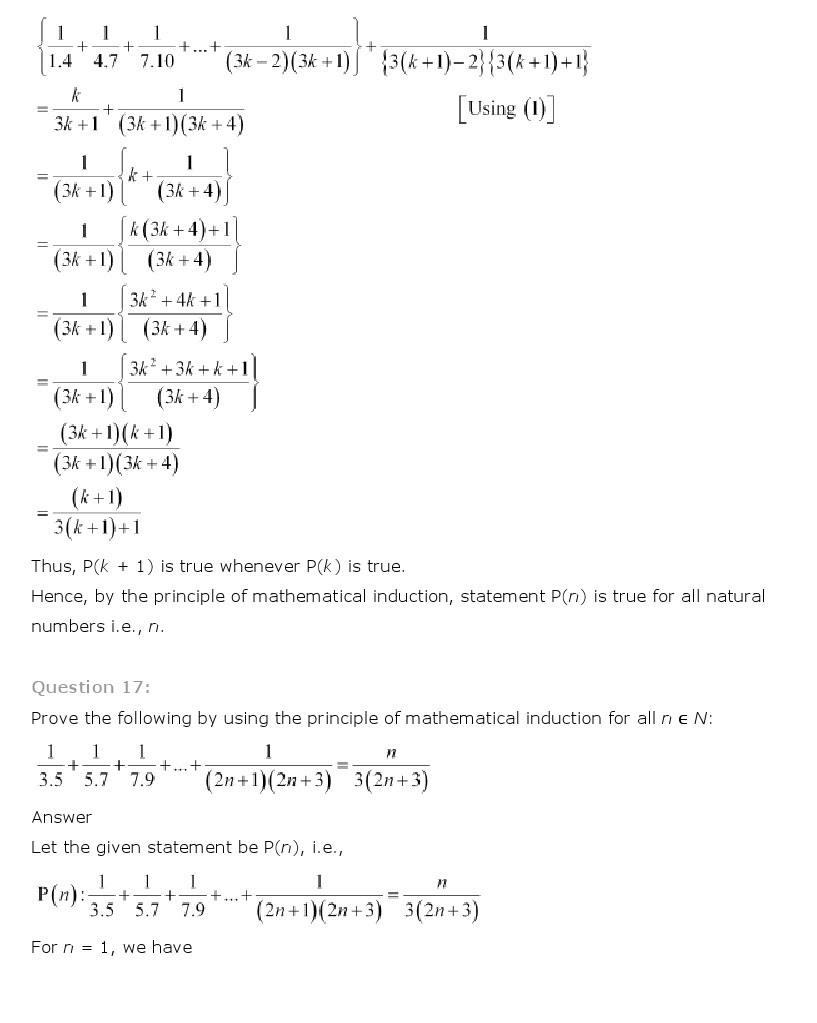## NCERT Solutions for Class 11th Maths Chapter 4 – Principle of Mathematical Induction

National Council of Educational Research and Training (NCERT) Book Solutions for class 11th
Subject: Maths
Chapter: Chapter 4 – Principle of Mathematical Induction
Class 11th Maths Chapter 4 Principle of Mathematical Induction NCERT Solution is given below.<< Back to NCERT/CBSE Notes